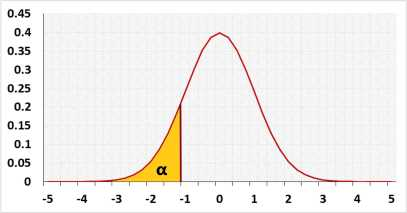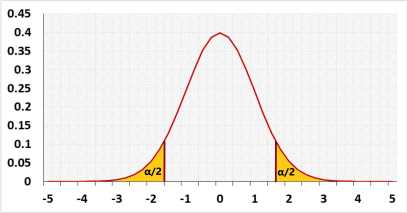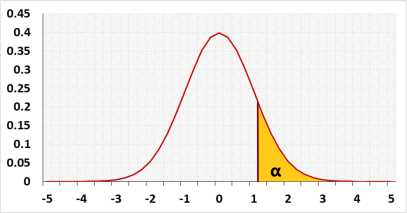# Proportion Sample Size Calculator

## Information

Calculate the sample size to gain the required test power and draw a power analysis chart.

### Effect

When you choose the Effect the tool determines the Effect size.
Ignore this field if you know the required Effect size.
If you do not know what to do, use the Medium effect.

You may also calculate the required effect size using the "Calculate h".
Example
The null assumption is that the population proportion is 0.7.
You suspect that the proportion is larger. You want to plan research that will reject the null assumption if the population proportion is 0.75 or larger.
1. Press the "Calculate h" button to open the calculation window.
2. Enter P1 = 0.7 and P2 = 0.75.
3. The calculator will update "h effect size" field with h effect size (0.11208).

### Relevant statistical tests

Use this calculator to choose the sample size of one of the following tests:
One Sample proportion Test
Two Sample proportion Test

### Example:

Two-tailed one-sample proportion test, α=0.05, power=0.8.
The test needs to identify a medium effect size: h=0.5.
Since the sample size is discrete you can not gain an exact power of 0.8.
A sample of n = 31 gains the power of 0.7950.
A sample of n = 32 gains the power of 0.8074.
Since the required test power is at least 0.8, we choose n = 32.

### Two-tailed